Choose Language Hide Translation Bar
Highlighted

## Test to identify which variable has the largest effect on measurement

I'm trying to figure out which of these variables:

 Person Fuel Sample Set

affects the measurement "Time" the most.

However the time will be different depending on the material used, so I think the analysis has to be done per material.

I tried a 3-factor anova: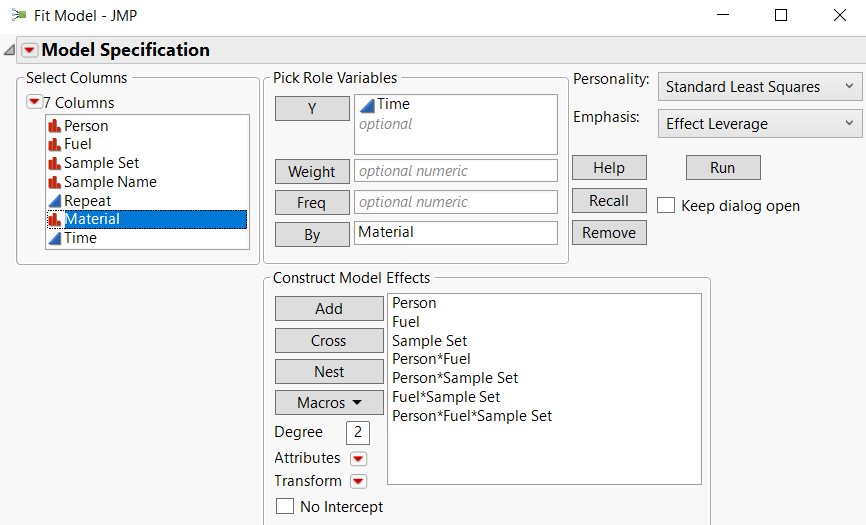However under effects, it just says LostDFs. Either because there are not enough points or because there are combinations missing.

For each material I have 54 data points (3 repeats of each for each sample)

All of the variables I'm investigating are binary, so 2*2*2 = 8 combinations, but I only have 6 combinations:

Alice 1 A    |    Bob 1 A

Alice 1 B    |    Bob 2 A

Alice 2 B    |    Bob 2 B

And am missing:

Alice 2 A    |    Bob 1 B

Which would be the best test to try and identify which of

 Person Fuel Sample Set

has the biggest effect on time?

Thank you

1 ACCEPTED SOLUTION

Accepted Solutions
Highlighted

## Re: Test to identify which variable has the largest effect on measurement

1. If you look at the file you posted, the Time column is set to nominal.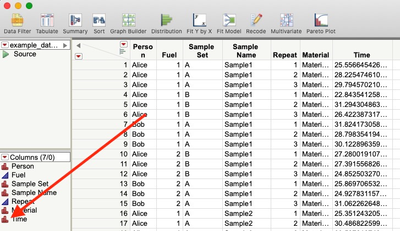2. Without knowing practical significance, it is impossible to analyze the data.

3. By normalizing you are accounting for the "expected" differences between materials.  You could try deviation from target or mean.

4. The repeats are not considered independent events and therefore are not additional degrees of freedom.  You can look at the individual data points graphically fords, and if there are no outliers, then summarizing those data points is appropriate to analyze the treatments.

5. I can't understand how a sample from one material can be "identical" to a sample from another material.  This is impossible.

4 REPLIES 4
Highlighted

## Re: Test to identify which variable has the largest effect on measurement

Here are my thoughts:

1. Your Time column is set as nominal, but I believe it is continuous.

2. How much of a change in the Time values is of practical significance?

3. If there is a known amount of time difference between materials, you could either do the analysis by material or normalize the data (delta from target for each material)

4. You should evaluate the repeated measures within treatment before summarizing that data.  Here is a range chart showing those measures are consistent, so summarizing this data with a mean (and standard deviation) is appropriate.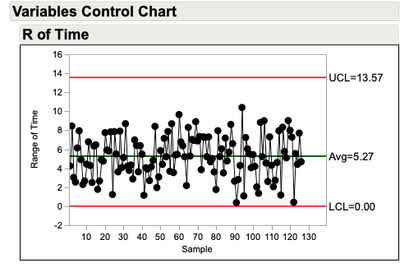5.  A questions about your data; You use the same designation for Sample Name across multiple Materials?  It doesn't seem likely that Sample1 for Material1 is identical to Sample1 for Material2?  These may be nested. Lost DF's is usually be cause you have over specified the model given the number of data points.  It also looks like there is a bit of imbalance to your data set?  If you have 7 materials, 2 fuels, 2 people, 2 sample sets and 3 sample names per material and 3 repeats, you should have 504 data points?

A bit of a look at the data: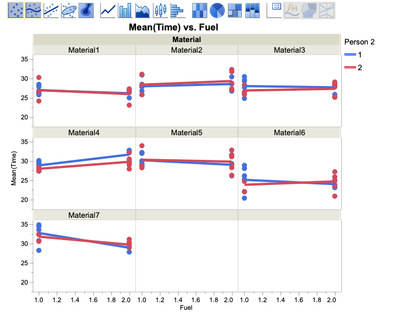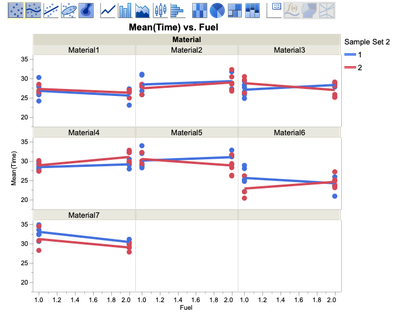Highlighted

## Re: Test to identify which variable has the largest effect on measurement

1. I believe the time column is set as continuous, not nominal.

2. Not sure on how much of a change is of practical significance, however I will be rounding to 2dp - the data has been modified for confidentiality.

3. I'm still trying to figure out how to normalise in order to be able to compare across materials but haven't been able to yet.

4. I'm not sure I understand this point, do you mean to replace the three repeats by the mean and sd of the three?

5. Yes, the samples are identical. And yes I did mention the imbalance, for every material and sample I am missing this combinations:

Alice 2 A    and    Bob 1 B

Highlighted

## Re: Test to identify which variable has the largest effect on measurement

1. If you look at the file you posted, the Time column is set to nominal.2. Without knowing practical significance, it is impossible to analyze the data.

3. By normalizing you are accounting for the "expected" differences between materials.  You could try deviation from target or mean.

4. The repeats are not considered independent events and therefore are not additional degrees of freedom.  You can look at the individual data points graphically fords, and if there are no outliers, then summarizing those data points is appropriate to analyze the treatments.

5. I can't understand how a sample from one material can be "identical" to a sample from another material.  This is impossible.

Highlighted

## Re: Test to identify which variable has the largest effect on measurement

Apologies, I don't know what happened to the time column, should have been numerical, you're right.

I think I need to discuss further with the team. I had forgotten about repeats not adding DoF which is what I need to look at.

Regarding samples being the same, it is the sample (of which there are only 3) being added to the material, not a sample from the material.

You've been very helpful. Thank you

Article Labels